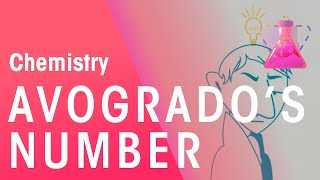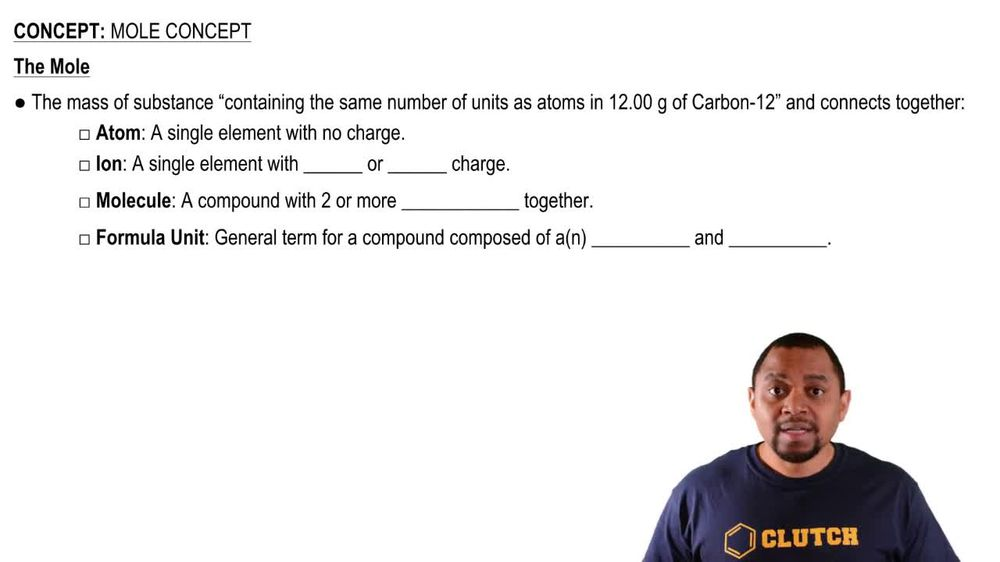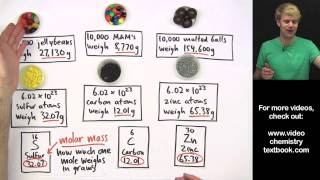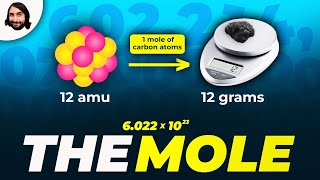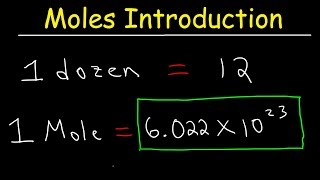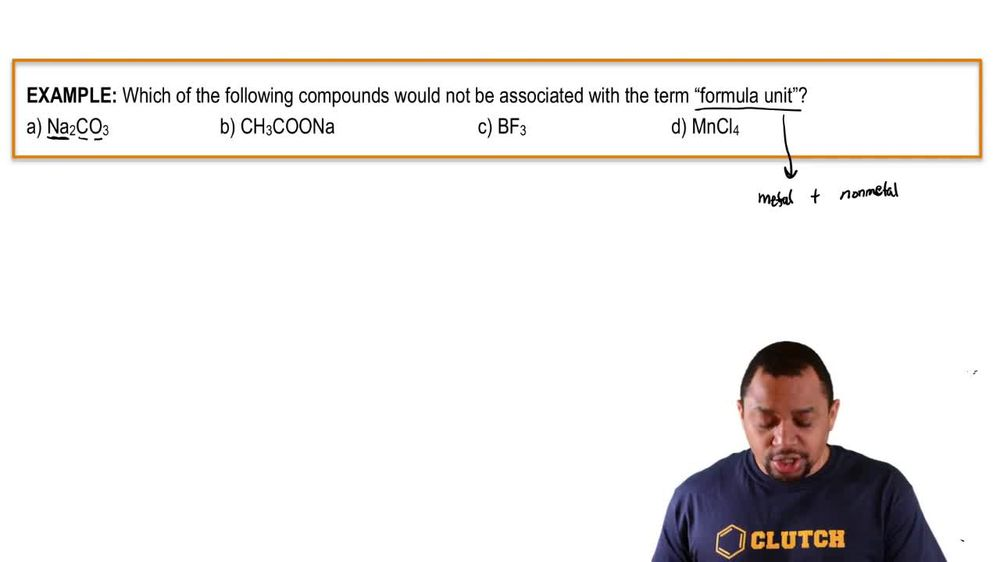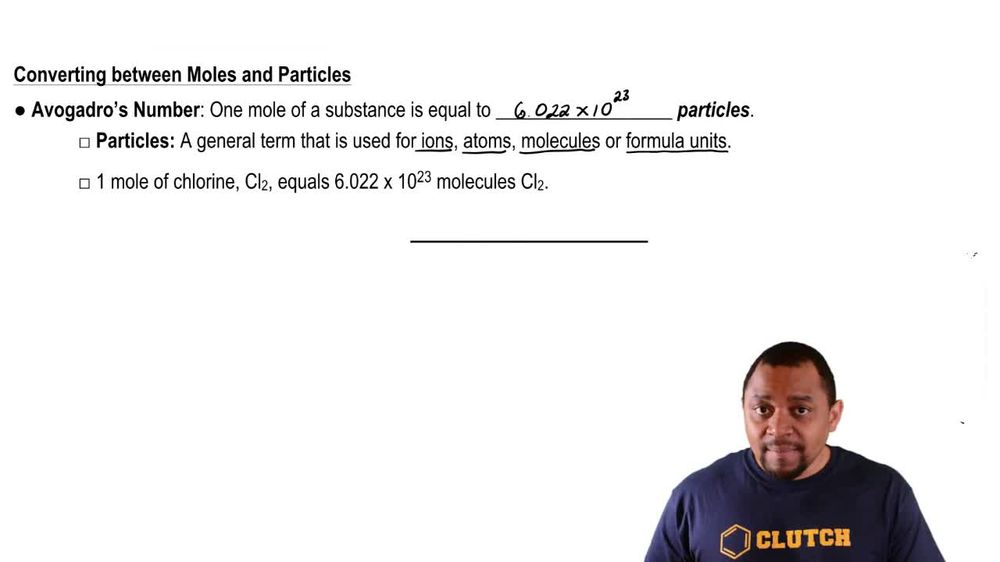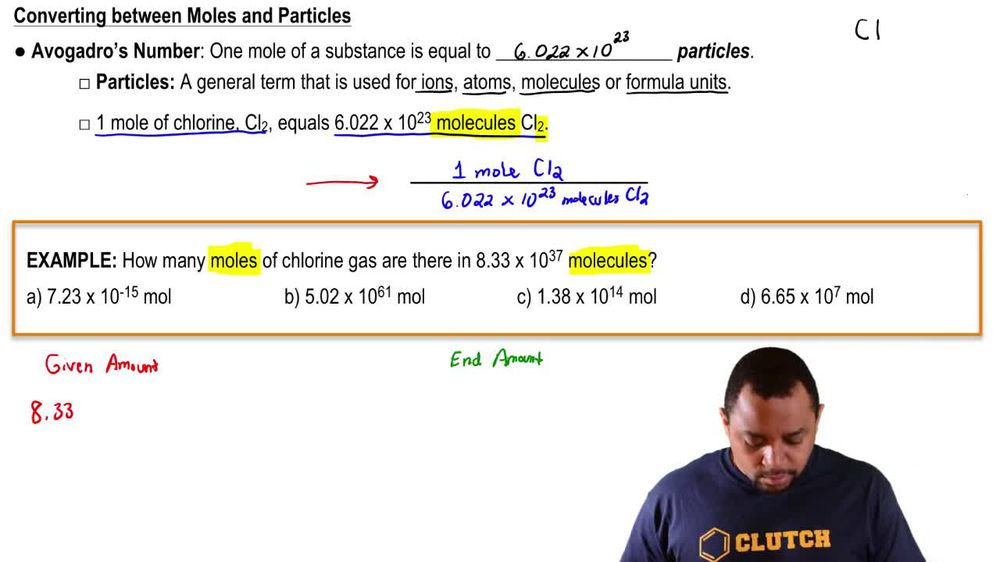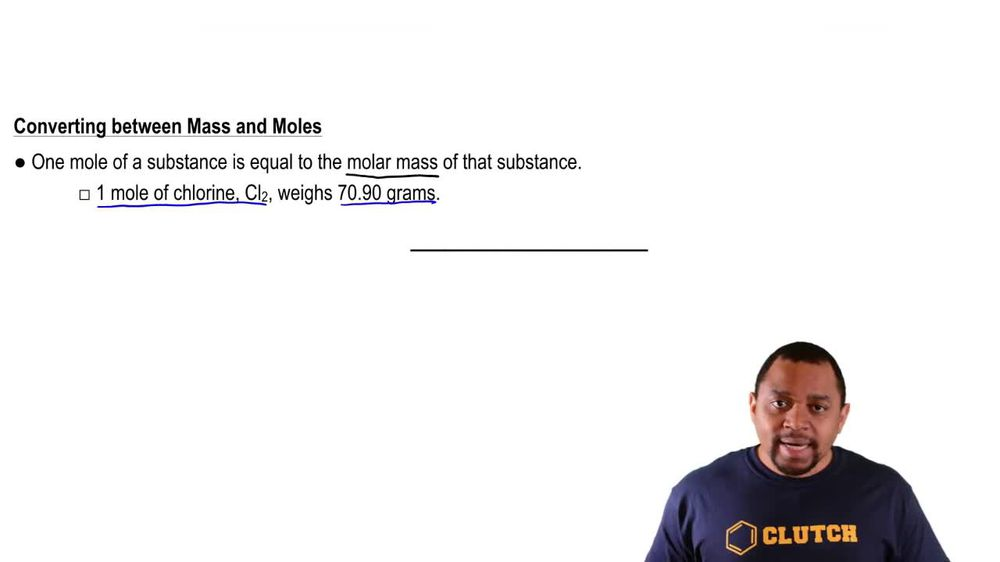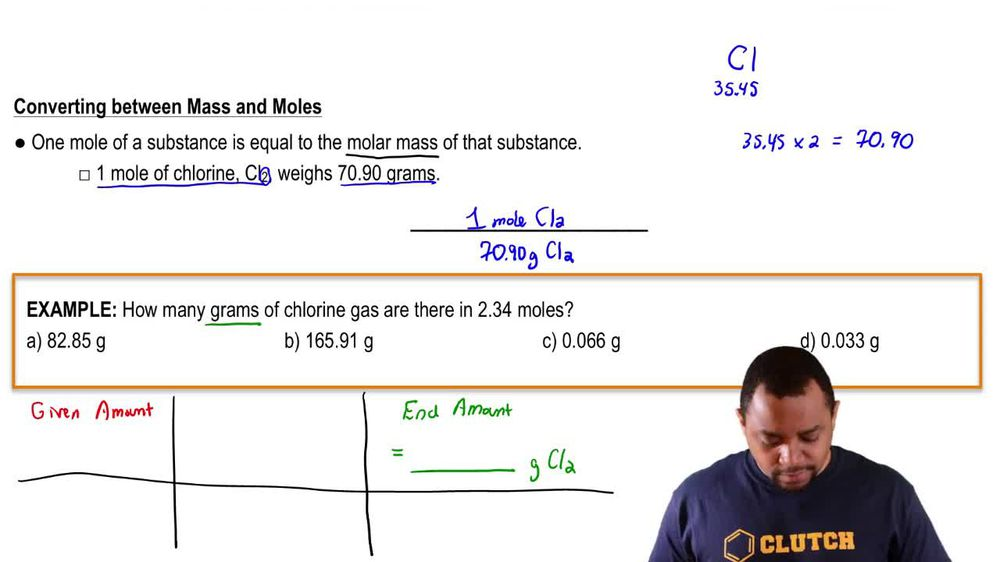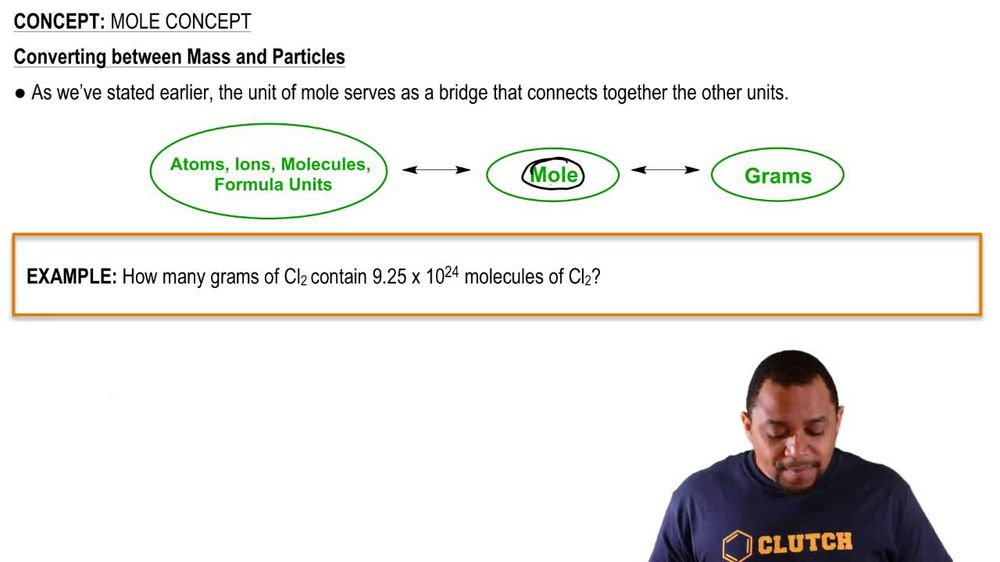Start typing, then use the up and down arrows to select an option from the list.
1. 2. Atoms & Elements2. Mole Concept
Problem

# What is the mass, in grams, of each elemental sample? a. 2.3 * 10 - 3 mol Sb

Relevant Solution48s
Play a video:
Hello everyone in this question, we asked, calculate the mass of 6.59 moles of copper in milligrams, The regular 6.59 moles of copper. And in order to convert to grams of copper, we need to use the molar mass of copper Molar mass is going to be .55 g and one mole of copper. And now to convert two mg, we have one g Equal to 1000 milligrams, And this gives us 4.19 Times 10 to the five milligrams hopper. Thanks for watching my video and I hope it was helpful.